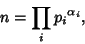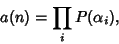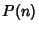## Kronecker Decomposition Theorem

Every Finite Abelian Group can be written as a Direct Product of Cyclic Groups of Prime Power Orders. In fact, the number of nonisomorphic Abelian Finite Groupsof any given Orderis given by writingaswhere theare distinct Prime Factors, thenwhereis the Partition Function. This gives 1, 1, 1, 2, 1, 1, 1, 3, 2, ... (Sloane's A000688).

Sloane, N. J. A. Sequence A000688/M0064 in An On-Line Version of the Encyclopedia of Integer Sequences.'' http://www.research.att.com/~njas/sequences/eisonline.html and Sloane, N. J. A. and Plouffe, S. The Encyclopedia of Integer Sequences. San Diego: Academic Press, 1995.